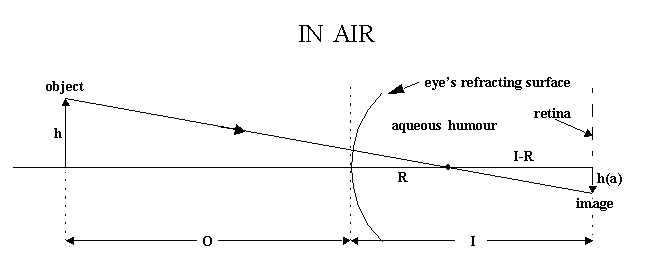# Do Things Seem 33% Bigger Underwater?

Not quite. Refraction at a planar water-air interface (such as due to a mask faceplate) produces an image that seems larger than the corresponding object, but the degree of magnification depends on both the object-to-faceplate and eye-to-faceplate distances. If the index of refraction of the water is n(w)=1.33 and that of air is n(a)=1.00, the factor 1.33 is the maximum possible magnification that can be produced by a planar water-air interface.`             h(a) = h(I-R)/(O+R)                             Equation 1``             h(w)=(I-R)tan(r)                                Equation 2`

`             h = Dtan(i) + (d+R)tan(r)                       Equation 3`

`             h(w)/h(a) = (O+R)/[Dtan(i)/tan(r) + (d+R)]      Equation 4`

`             sin(i)/sin(r) = n(a)/n(w)                       Equation 5`

`             tan(i)/tan(r) = n(a)/n(w)                       Equation 6`

`             M = h(w)/h(a) = (D+d+R)/[Dn(a)/n(w) + (d+R)]    Equation 7`

`             M =m{[ (1+ (d+R)/D]/[1+m(d+R)/D]}               Equation 8`

`             m = n(w)/n(a) = 1.33                            Equation 9`

```      object to   eye to
faceplate  faceplate
D (cm)     d (cm)       M
-----------------------------
200         0        1.328
100         0        1.327
50         0        1.323
200        2.5       1.323
100        2.5       1.316
50        2.5       1.303
25        2.5       1.281
100        5.0       1.306
50        5.0       1.286
25        5.0       1.252
```

The foregoing dealt strictly with the size of the retinal image. How the brain perceives that image depends on many other factors. Studies have shown (Ross et al), that the brain adapts rather quickly to the underwater environment, so that initial errors in judging object sizes are significantly reduced during the course of a dive. Moreover, size judgments improve with diving experience.

REFERENCES:

H. E. Ross, S. S. Franklin, G. Weltman, and P. Lennie, 'Adaptation of Divers to Size Distortion Under Water', Br. J. Psychol. (1970), 61, pp. 365-373.